# Hexbin map in R: an example with US states

This post describes how to build a hexbin map. It is based on a `geojson` file providing the boundaries of us states as hexagons.

# Basic hexbin map

The first step is to build a basic hexbin map of the US. Note that the gallery dedicates a whole section to this kind of map.

Hexagones boundaries are provided here. You have to download it at the `geojson` format and load it in R thanks to the `geojson_read()` function. You get a geospatial object that you can plot using the `plot()` function. This is widely explained in the background map section of the gallery.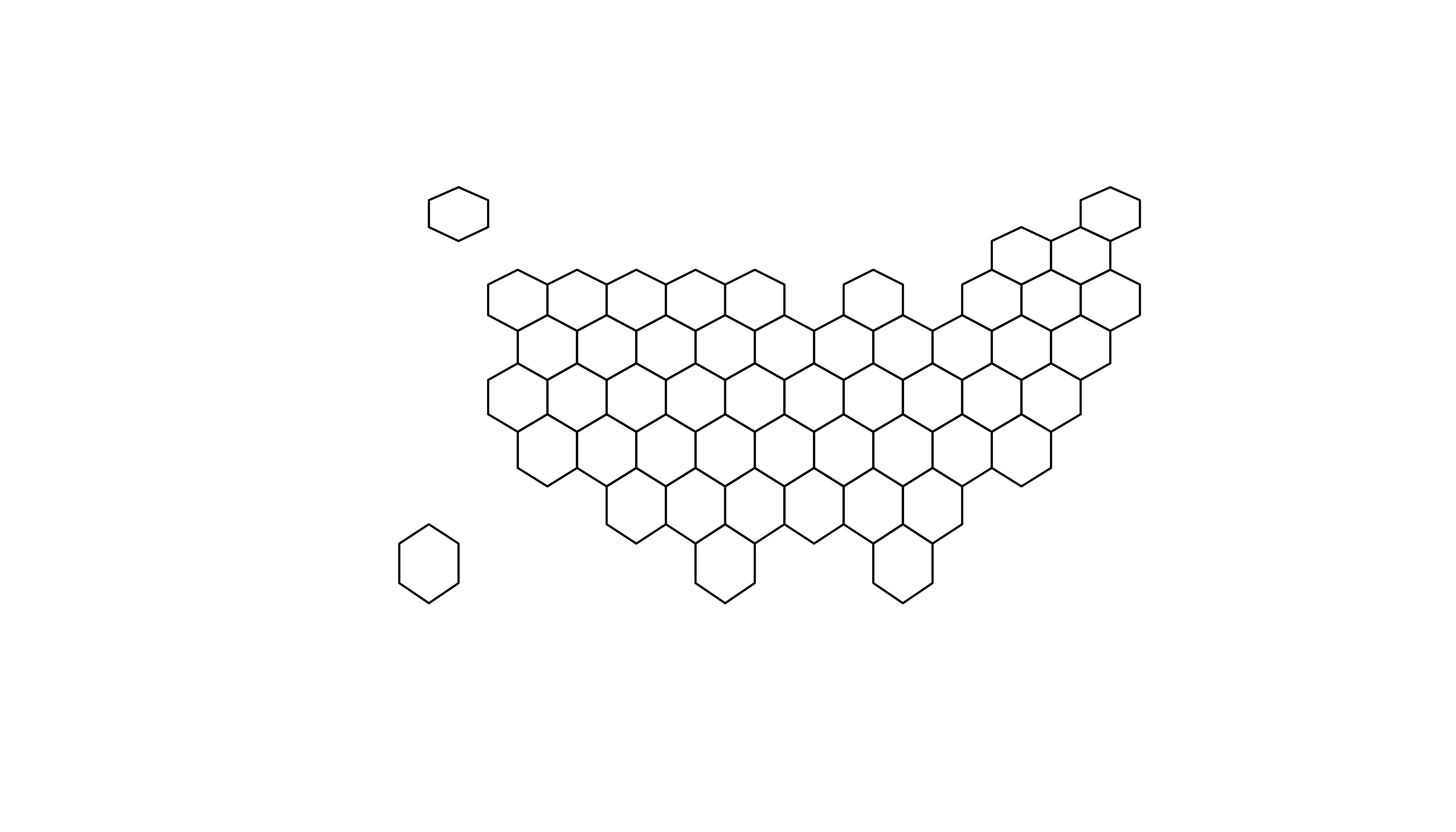``````# library
library(tidyverse)
library(geojsonio)
library(RColorBrewer)
library(rgdal)

# Load this file. (Note: I stored in a folder called DATA)
spdf <- geojson_read("DATA/us_states_hexgrid.geojson.json",  what = "sp")

# Bit of reformating
spdf@data = spdf@data %>%

# Show it
plot(spdf)``````

# `ggplot2` and state name

It is totally doable to plot this geospatial object using `ggplot2` and its `geom_polygon()` function, but we first need to `fortify` it using the `broom` package.

Moreover, the `rgeos` package is used here to compute the centroid of each region thanks to the `gCentroid` function.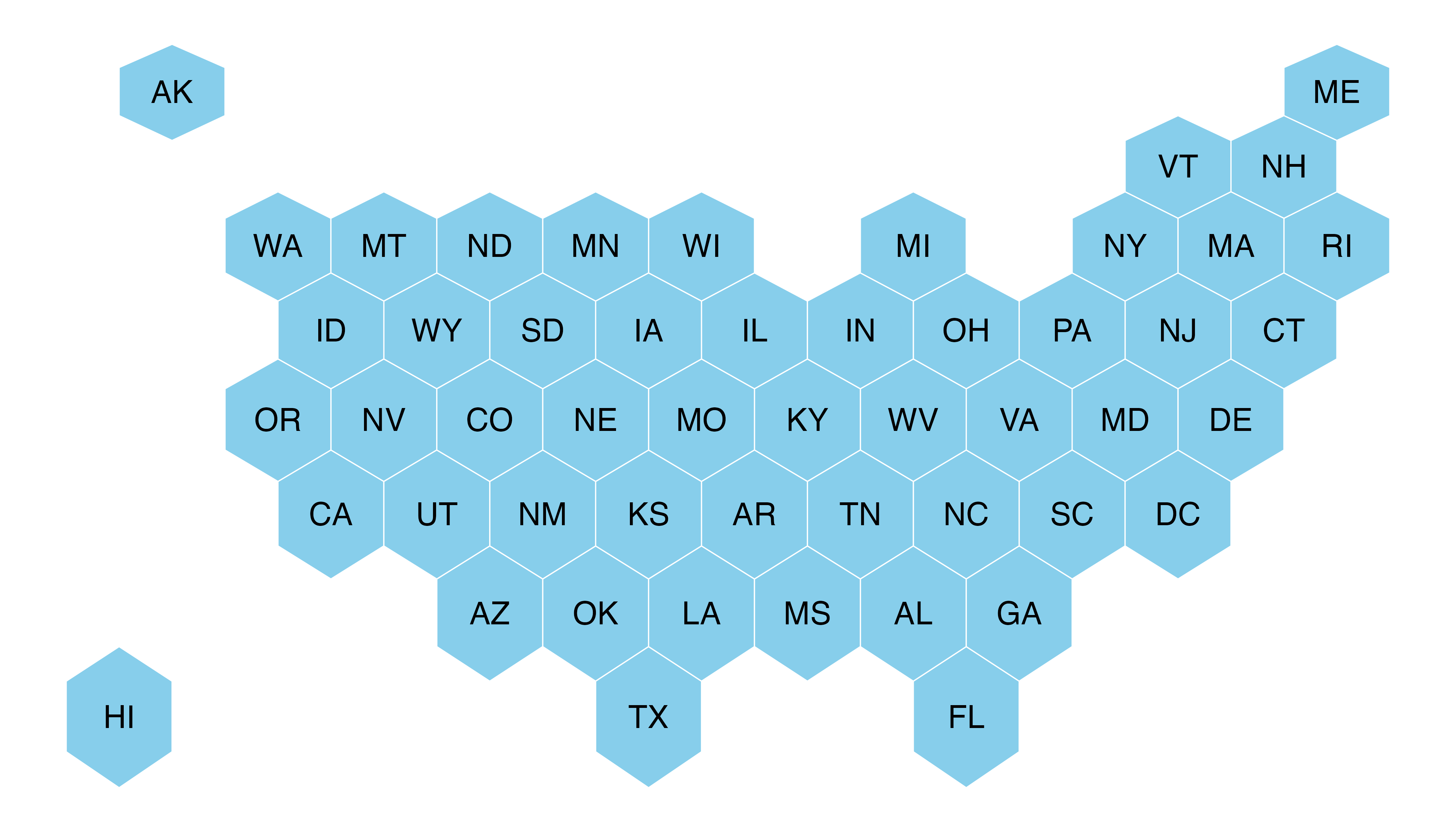``````# I need to 'fortify' the data to be able to show it with ggplot2 (we need a data frame format)
library(broom)
spdf_fortified <- tidy(spdf, region = "google_name")

# Calculate the centroid of each hexagon to add the label:
library(rgeos)
centers <- cbind.data.frame(data.frame(gCentroid(spdf, byid=TRUE), id=spdf@data\$iso3166_2))

# Now I can plot this shape easily as described before:
ggplot() +
geom_polygon(data = spdf_fortified, aes( x = long, y = lat, group = group), fill="skyblue", color="white") +
geom_text(data=centers, aes(x=x, y=y, label=id)) +
theme_void() +
coord_map()``````

# Basic choropleth

Now you probably want to adjust the color of each hexagon, according to the value of a specific variable (we call it a choropleth map).

In this post I suggest to represent the number of wedding per thousand people. The data have been found here, and stored on a clean format here.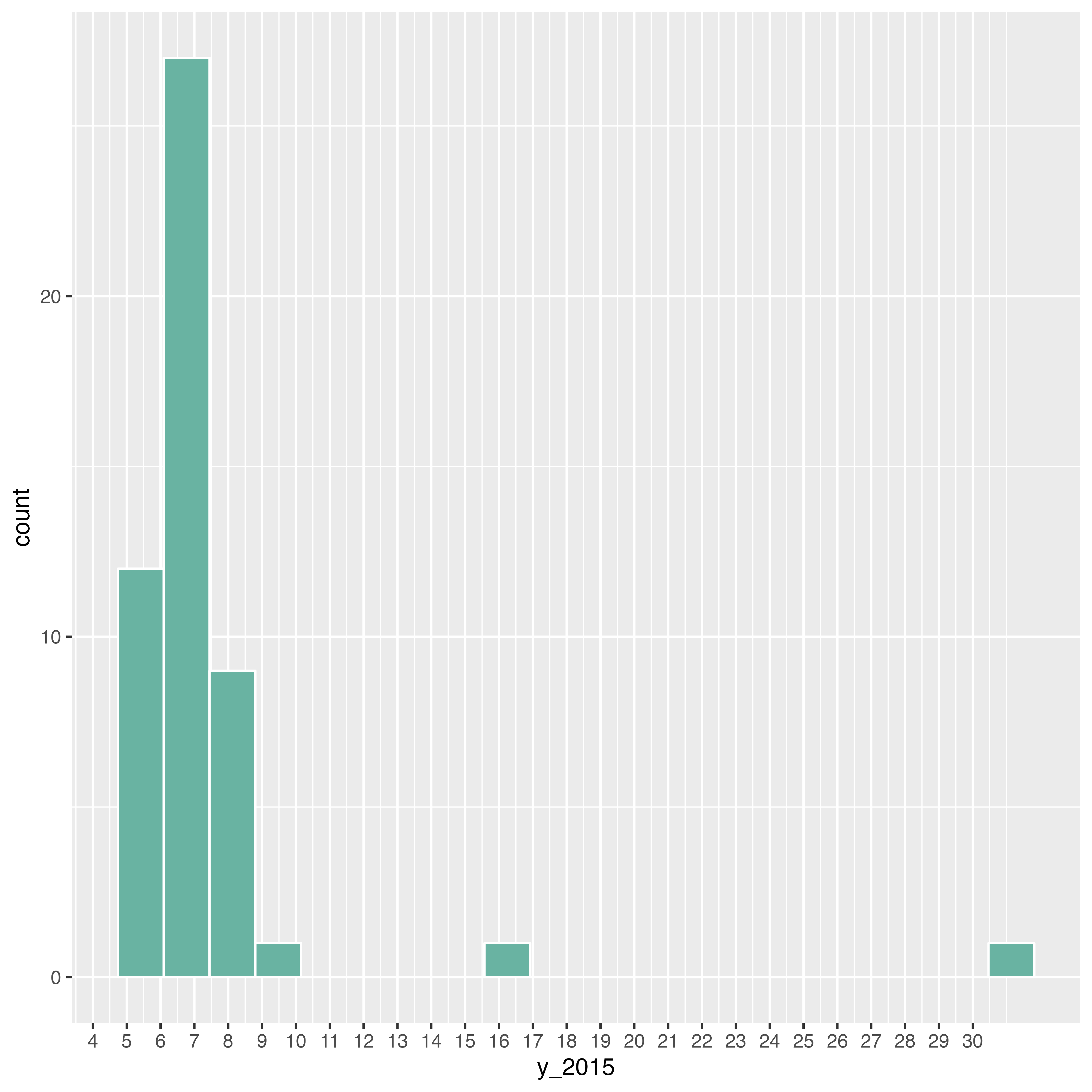``````# Load mariage data

# Distribution of the marriage rate?
data %>%
ggplot( aes(x=y_2015)) +
geom_histogram(bins=20, fill='#69b3a2', color='white') +
scale_x_continuous(breaks = seq(1,30))``````

Most of the state have between 5 and 10 weddings per 1000 inhabitants, but there are 2 outliers with high values (16 and 32).

Let’s represent this information on a map. We have a column with the state id in both the geospatial and the numerical datasets. So we can merge both information and plot it.

Note the use of the `trans = "log"` option in the color scale to decrease the effect of the 2 outliers.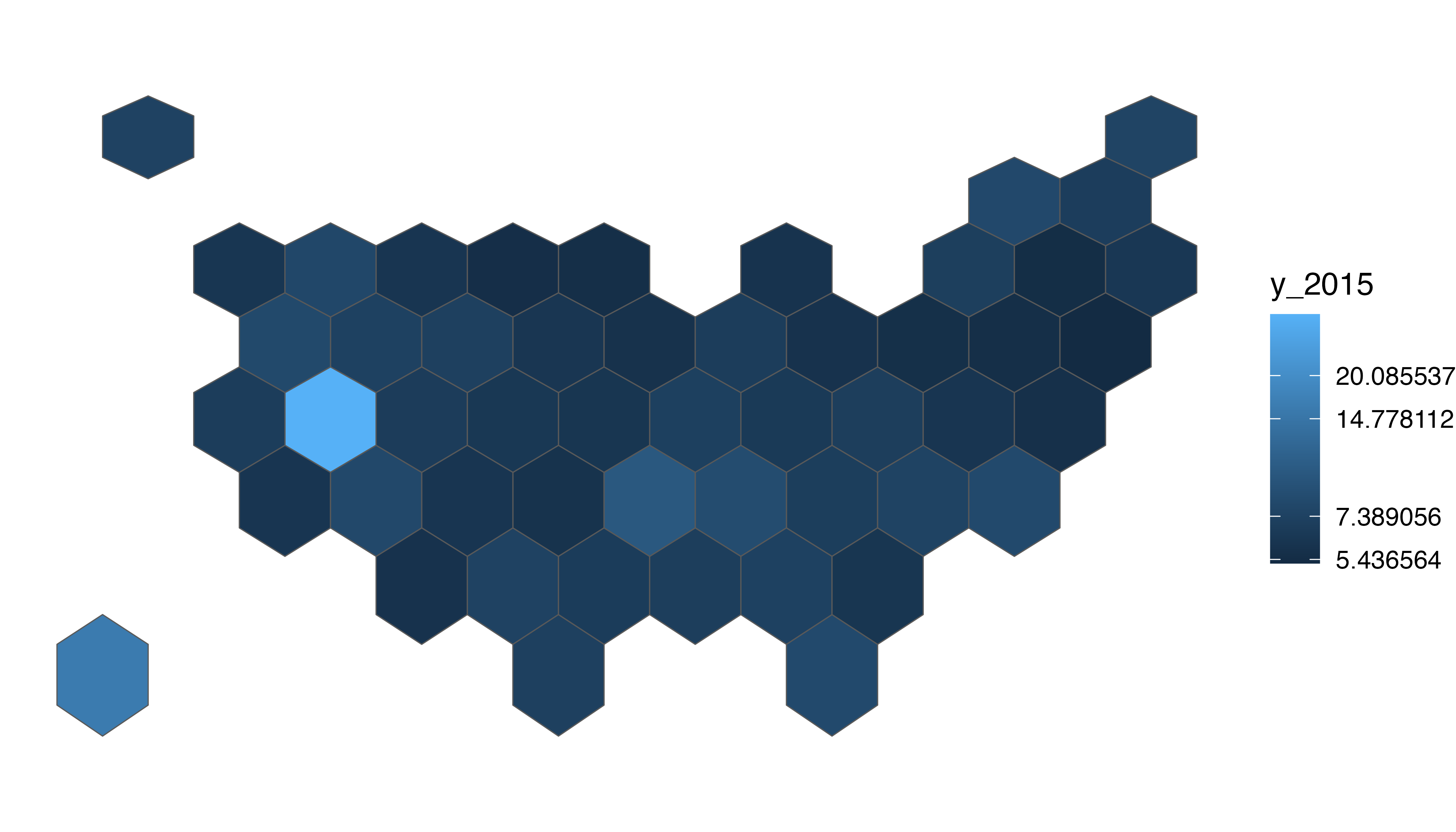``````# Merge geospatial and numerical information
spdf_fortified <- spdf_fortified %>%
left_join(. , data, by=c("id"="state"))

# Make a first chloropleth map
ggplot() +
geom_polygon(data = spdf_fortified, aes(fill =  y_2015, x = long, y = lat, group = group)) +
theme_void() +
coord_map()``````

# Customized hexbin choropleth map

Here is a final version after applying a few customization:

• Use handmade binning for the colorscale with `scale_fill_manual`
• Use `viridis` for the color palette
• Add custom legend and title
• Change background color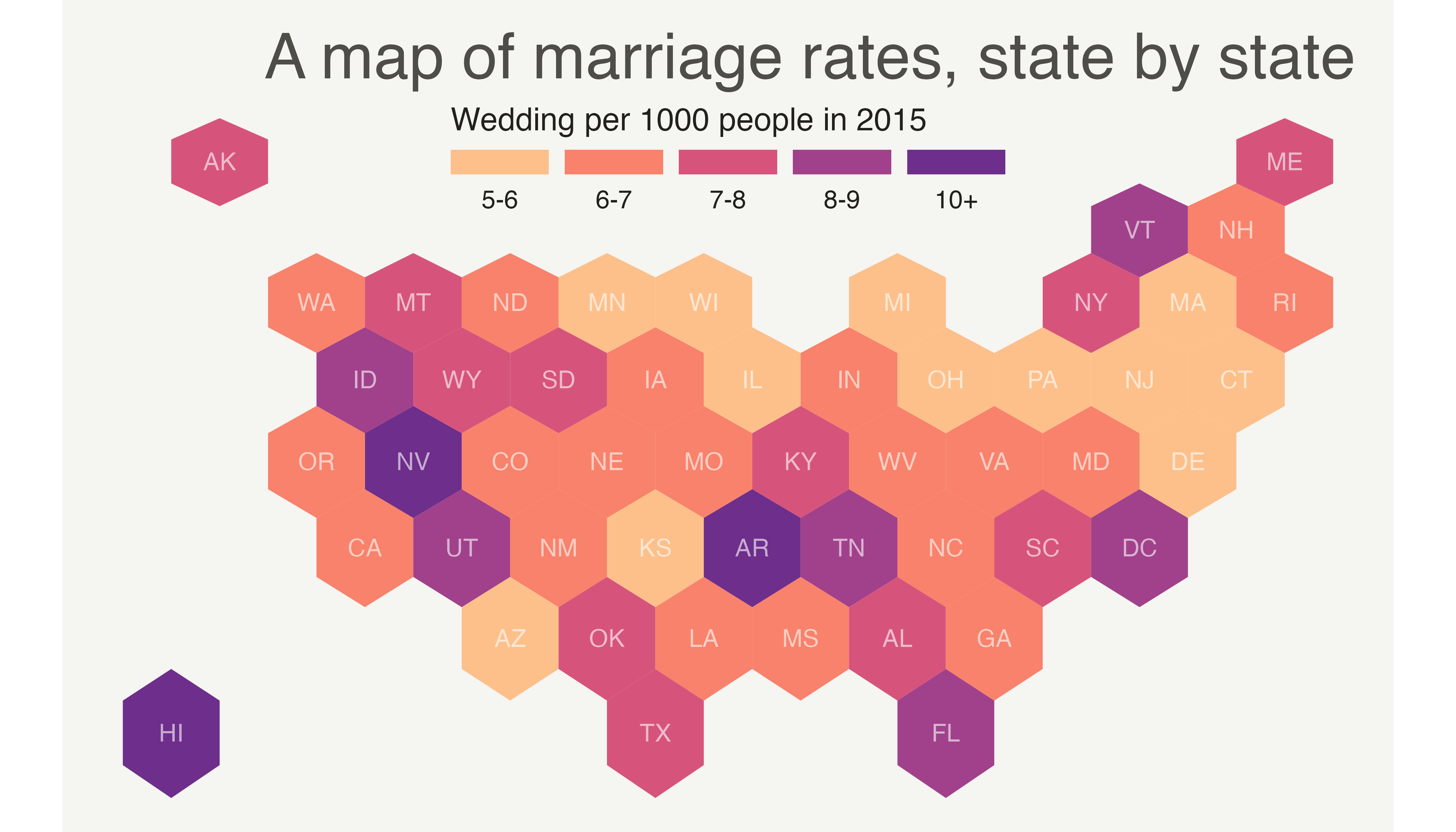``````# Prepare binning
spdf_fortified\$bin <- cut( spdf_fortified\$y_2015 , breaks=c(seq(5,10), Inf), labels=c("5-6", "6-7", "7-8", "8-9", "9-10", "10+" ), include.lowest = TRUE )

# Prepare a color scale coming from the viridis color palette
library(viridis)
my_palette <- rev(magma(8))[c(-1,-8)]

# plot
ggplot() +
geom_polygon(data = spdf_fortified, aes(fill = bin, x = long, y = lat, group = group) , size=0, alpha=0.9) +
geom_text(data=centers, aes(x=x, y=y, label=id), color="white", size=3, alpha=0.6) +
theme_void() +
scale_fill_manual(
values=my_palette,
name="Wedding per 1000 people in 2015",
guide = guide_legend( keyheight = unit(3, units = "mm"), keywidth=unit(12, units = "mm"), label.position = "bottom", title.position = 'top', nrow=1)
) +
ggtitle( "A map of marriage rates, state by state" ) +
theme(
legend.position = c(0.5, 0.9),
text = element_text(color = "#22211d"),
plot.background = element_rect(fill = "#f5f5f2", color = NA),
panel.background = element_rect(fill = "#f5f5f2", color = NA),
legend.background = element_rect(fill = "#f5f5f2", color = NA),
plot.title = element_text(size= 22, hjust=0.5, color = "#4e4d47", margin = margin(b = -0.1, t = 0.4, l = 2, unit = "cm")),
)``````

Related chart types

## Contact

This document is a work by Yan Holtz. Any feedback is highly encouraged. You can fill an issue on Github, drop me a message on Twitter, or send an email pasting yan.holtz.data with gmail.com.